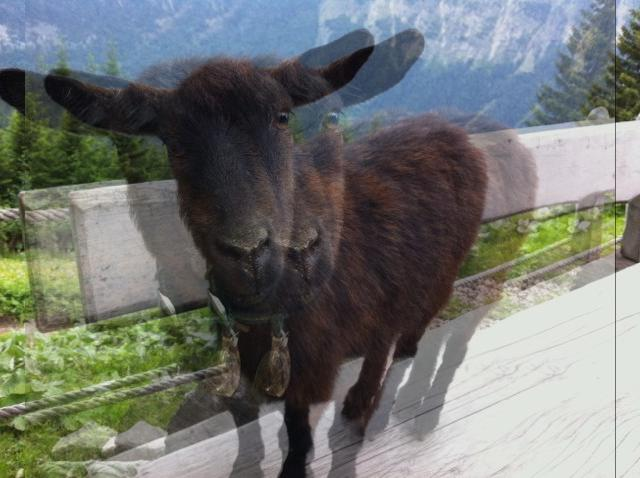# pheelicks

This post is part of a series. For a listing of all the posts, as well as instructions on running the code, see here.

Recently I came across a most agreeable app, Instagram, that lets you add filters and what-not to your photos, and I thought it would be remiss not to try something similar in Go. And while we’re at it, why not introduce the concept of Interfaces.

## Goat

Let me introduce you to a sweet goat.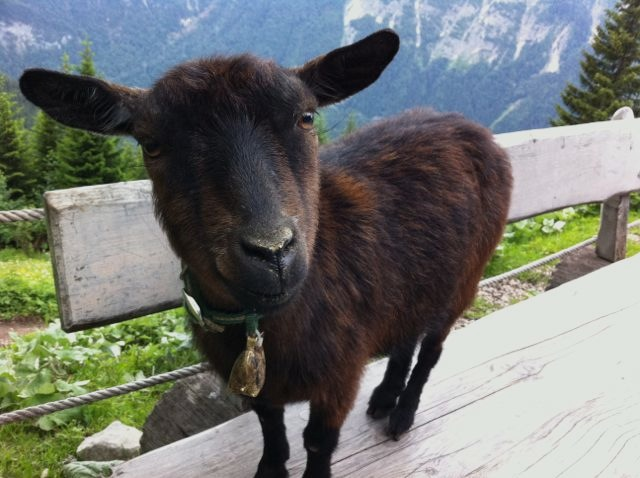Personally, I like this picture as it is, but I’m told to that to appeal to Generation Z, we need to apply a filter before it is deemed worthy for wider circulation. A blur filter seems the obvious choice.

## Blur

At a high level, blurring an image consists of, for each pixel `p`:

1. Retrieving the neighbouring pixels in the vicinity of `p` based on some criteria, e.g. pixels no more than 5 pixels away from `p`
2. Combining the values from the neighbouring set of pixels, assigning a weight to each pixel based on some function, e.g. the further away we are from `p`, the lower the weight
3. Normalising our value, by diving by the sum of the weights used in the previous step
4. Assigning the normalised value to the corresponding pixel in the blurred image

For now, let’s not worry about the weighting function, and just assign each neighbouring pixel the same weight - also known as Box Blur. This will allow us to focus on the underlying algorithm more easily.

## Box Blur

We’ll want to extend the Canvas type with two new methods, `Blur` and `BlurPixel`. The first will actually perform the blurring on the image, while the second is used as a helper to calculate the blurred value for a single pixel.

`Blur` is pretty straightforward, it creates a copy of the canvas, then fills it in, pixel by pixel, by applying the `BlurPixel` function to each pixel in the original canvas:

``````func (c Canvas) Blur(radius int) {
clone := c.Clone()
size := c.Bounds().Size()
for x := 0; x < size.X; x++ {
for y := 0; y < size.Y; y++ {
clone.Set(x, y, color)
}
}
copy(c.Pix, clone.Pix)
}
``````

`BlurPixel` is more interesting:

``````func (c Canvas) BlurPixel(x int, y int, radius int) color.Color {
weightSum := float64(0)
size := c.Bounds().Size()
outR, outG, outB := float64(0), float64(0), float64(0)
r, g, b, _ := c.At(i, j).RGBA()
outR += float64(r)
outG += float64(g)
outB += float64(b)
weightSum += 1.0
}
}
// Need to divide by 0xFF as the RGBA() function returns color values as uint32
// and we need uint8
return color.RGBA{
uint8(outR / (weightSum * 0xFF)),
uint8(outG / (weightSum * 0xFF)),
uint8(outB / (weightSum * 0xFF)),
255}
}
``````

This is more or less steps 2. and 3. that are listed above. Note that some edge case handling has been omitted for clarity. To see the function in full check out canvas.go

Applying this to our charming, yet long-suffering goat, we get: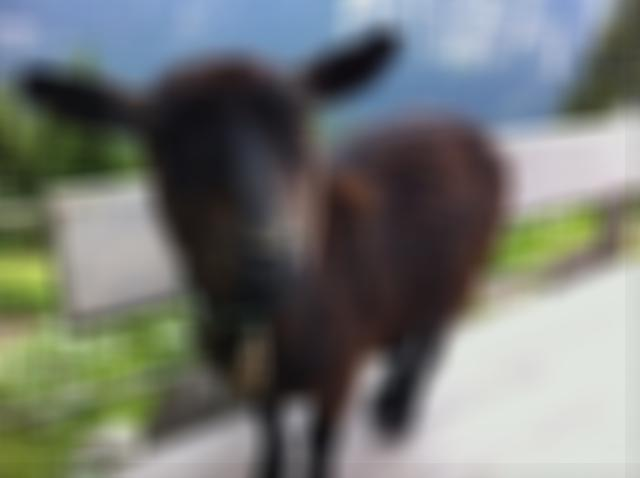One thing to note is that for a given `radius`, we need to retrieve `radius * radius` pixels when running BlurPixel, and as such the running time gets pretty slow for large values of `radius`. Anything under 10 should easily be fine though.

For an example of how to load in an image and apply the `Blur` function to, see the blur.go example on github.

## Interfaces and Weighting functions

It’s now time to add in a weighting function, to allow us to perform different types of blur effects. Given that the rest of of the blurring algorithm stays the same, it seems natural to pass the weighting type as a parameter in the BlurPixel method. To do this, we’ll define an `Interface` for what we expect our weighting type to do, and then our `BlurPixel` method will know what methods it can call on the type. Here goes:

``````type WeightFunction interface {
Weight(x int, y int) float64
}
``````

And the accompanying Box Blur implementation of this interface:

``````type WeightFunctionBox struct{}

func (w WeightFunctionBox) Weight(x int, y int) float64 { return 1.0 }
``````

To clarify, the `x` and `y` parameters passed to the `Weight` function are relative to the pixel being processed. So with this in hand we can modify our `BlurPixel` function to:

``````func (c Canvas) BlurPixel(x int, y int, radius int, weight WeightFunction) color.Color {
weightSum := float64(0)
size := c.Bounds().Size()
outR, outG, outB := float64(0), float64(0), float64(0)
weight := weight.Weight(i-x, j-y)
r, g, b, _ := c.At(i, j).RGBA()
outR += float64(r) * weight
outG += float64(g) * weight
outB += float64(b) * weight
weightSum += weight
}
}
// Rest of function...
``````

An important thing to note is that when defining our `WeightFunctionBox` type, nowhere did we explicitly state that we were implementing the `WeightFunction` interface. This is because in Go interfaces are satisfied implicitly, that is: any object which implements the methods of an interface, implements the interface. This puts Go somewhere between Java (where you have to explicitly implement an interface) and JavaScript (where you can pass whatever the hell you like around as there are no interfaces, but things will go wrong if your mystery object doesn’t implement a method that you expect it to).

To see how this all fits together into a working example, see the blur.go and canvas.go files on github.

## More weighting functions

If we pipe through the `weight` variable through to the `Blur` method, we can easily apply different types of blur to a `Canvas`, e.g.

``````canvas.Blur(5, new(WeightFunctionBox))
``````

We can now have some fun experimenting with different weighting functions. Here are a couple of examples, along with the results:

``````func (w WeightFunctionDist) Weight(x int, y int) float64 {
d := math.Hypot(float64(x), float64(y))
return 1 / (1 + d)
}
``````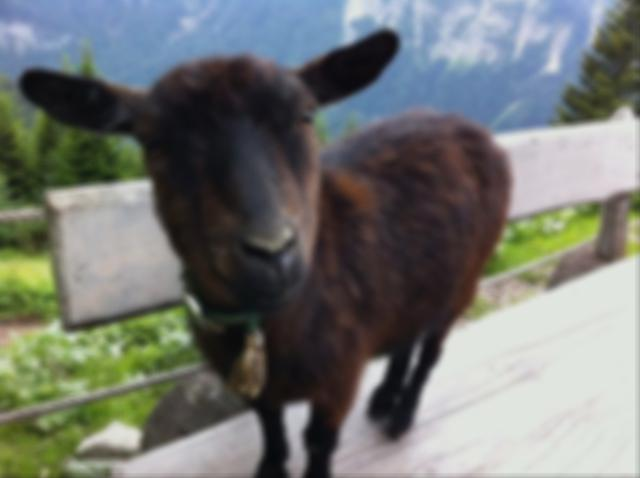``````func (w WeightFunctionMotion) Weight(x int, y int) float64 {
if y != 0 {
return 0
}
if x < 0 {
return 0
}
return 1 / (1 + float64(x))
}
``````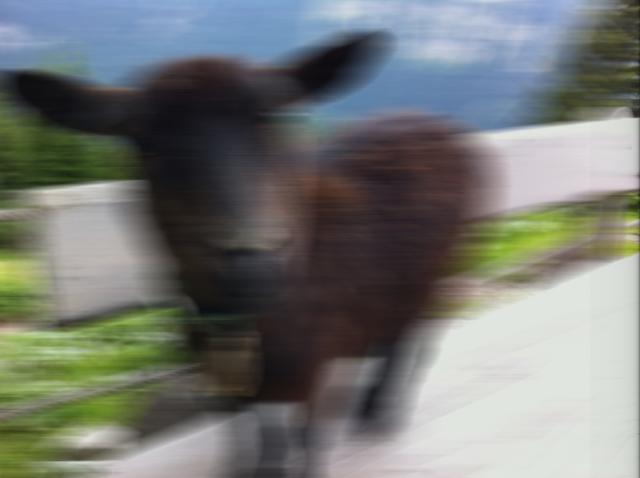``````type WeightFunctionDouble struct{
split int
}

func (w WeightFunctionDouble) Weight(x int, y int) float64 {
if y == 0 && (x == w.split || x == -w.split) {
return 1.0
} else {
return 0
}
}
``````